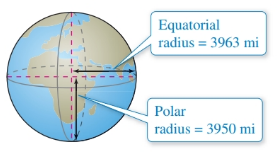Chapter 7.2, Problem 49E### Calculus: An Applied Approach (Min...

10th Edition
Ron Larson
ISBN: 9781305860919

#### Solutions

Chapter
Section### Calculus: An Applied Approach (Min...

10th Edition
Ron Larson
ISBN: 9781305860919
Textbook Problem
71 views

# Physical Science Because of the forces caused by its rotation, Earth is an oblate ellipsoid rather than a sphere. The equatorial radius is 3963 miles and the polar radius is 3950 miles. Find an equation of the ellipsoid. (Assume that the center of Earth is at the origin and that the trace formed by the plane z = 0 corresponds to the equator.)To determine

To calculate: The equation of oblate ellipsoid shape of the Earth where the equatorial radius and polar radius of the Earth are 3963 miles and 3950 miles respectively and the centre of the Earth is at origin and trace formed by the plane z=0 corresponds to the equator.

Explanation

Given Information:

The equatorial radius and polar radius of the Earth are 3963 miles and 3950 miles respectively and the centre of the Earth is at origin and trace formed by the plane z=0 corresponds to the equator.

Formula used:

The standard equation of oblate ellipsoid is,

x2a2+y2b2+z2c2=1

wherea,b,careinterceptonx,y,zaxisrespectively

Calculation:

The standard form of equation of an ellipsoid for centre (0,0,0) is,

x2a2+y2b2+z2c2=1

Since, the trace of the Earth formed by the plane z=0 at the equator that means the z axis of the Earth must passes through the polar of the Earth.

The intercept on the z axis is c that means c is the polar radius of the Earth.

c=3950 miles

Now, the oblate ellipsoid is a type of ellipsoid in which the two intercepts are equal

### Still sussing out bartleby?

Check out a sample textbook solution.

See a sample solution

#### The Solution to Your Study Problems

Bartleby provides explanations to thousands of textbook problems written by our experts, many with advanced degrees!

Get Started

#### In Exercises 29-34, rationalize the denominator of each expression. 31. 1xy

Applied Calculus for the Managerial, Life, and Social Sciences: A Brief Approach

#### In Problems 7-34, perform the indicated operations and simplify. 29.

Mathematical Applications for the Management, Life, and Social Sciences

#### The directrix of the conic given by is:

Study Guide for Stewart's Multivariable Calculus, 8th